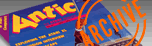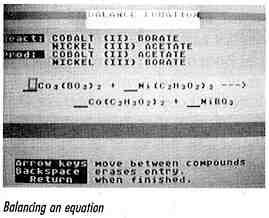`ANTIC VOL. 8, NO. 7 / DECEMBER 1989`

TRIPLE DISK BONUS

# Chemistry Tutor

Learn your ions, stoichiometry and balanced equations.

By John KennedyIn the April, 1989 issue of Antic we printed Periodic Madness, which drilled students about chemical elements, their symbols and atomic masses as shown on the Periodic Table of the Elements. Now, John Kennedy's Chemistry Tutor takes students several steps further, into the realm of ions, stoichiometry and balanced equations.

Chemistry Tutor is a BASIC program, but far too long for a type-in. The program can easily be RUN straight from the Antic Monthly Disk -- just press the number to the right of CHEMTUTR.BAS on the menu, and press [RETURN]. Your tutor will LOAD and RUN right away.

However, actually using the tutor won't be so easy, unless you already have a basic understanding of beginning chemistry and stoichiometry (proportional weights and measures involved in chemical activity,) and hence the science of balancing equations.

Author John Kennedy teaches Chemistry, Physics and Computer Programming at Northwestern High School near Springfield, Ohio. He developed the Chemistry Tutor to help his beginning chemistry students who had trouble writing and balancing equations and solving mass-mass problems. He says, "I wanted the program to provide a variety of questions and respond to incorrect answers with helpful suggestions. The program also had to be easy to use, even by students with little experience with computers." Thanks to the 8-bit Atari's graphics, the resulting program was both educational and colorful.

USING THE PROGRAM

After the title screen, a menu displays the following choices: Name Ions, Write Symbols and Charges for Ions, Write Chemical Formulas, Write Chemical Equations, Stoichiometric Calculations, and End Program. Pressing the [OPTION] key cycles through the choices. When the pointer is at the desired choice, press the [SELECT] key.

The first three choices each give a sequence of 10 questions, displaying a running total of right and wrong answers. Name Ions gives the ion's symbol and charge, and the user must name the ion. For example, the symbol ClO3 (charge -1) must be identified as CHLORATE.

The program uses the Stock system, in which elements with more than one positive oxidation state are identified by Roman numerals. For example, iron with a +2 oxidation number would be designated as Iron (II). Leave a space between the name and the parenthesis. If an incorrect answer is entered when naming or writing symbols for ions, the correct answer is displayed in the error window and your score is shown.

In Write Symbols and Charges for Ions, the program gives the name of and ion, and the user must enter the symbol and then the charge of that ion. Both must be correct to receive credit. If the symbol for CARBONATE is correctly entered as CO3, the user will then be asked for the charge -- in this case, -2.

Use a minus sign to designate negative charges. A plus sign for positive charges is optional. For this section, all numbers typed will appear as subscripts. Letters will be in lower case, with the [SHIFT] key used to produce capitals -- the abbreviations of the elements must be correctly capitalized.

FORMULAS & EQUATIONS

The three options on balancing equations and stoichiometry give one question at a time. Once a question has been completed, the program asks if you want to do another.

When the Write Chemical Formulas or the Write Chemical Equations options are selected, you will be asked to write the formulas for chemical compounds such as LITHIUM CYANATE (LiOCN) -- or to give the name from the formula.

Names for compounds are generated randomly from positive and negative ions. No spaces should be used when writing formulas. When an incorrect formula is entered, a hint will be displayed in the error window. After three unsuccessful attempts, the correct answer is displayed.

If you are writing equations, you will enter the formulas for two reactants and two products. When the complete equation is in the display window, a cursor will appear in front of the first compound. Typing numbers will produce standard numerals as coefficients. Use the [ARROW KEYS] to move from one compound to another until the equation is balanced. If you leave a blank space in front of any compound, it will be interpreted as a number 1.

If the equation is not balanced, a help screen will be shown in the information window. This screen displays the number of each ion as a reactant and as a product and if the two are in balance. Each time a coefficient is changed the display is updated. When the display shows a YES for each product and reactant, the equation is balanced. The coefficients must be reduced to their least possible values (like fractions) to be correct.

Stoichiometric Calculations first requires that an equation be written and balanced. A mass for one compound is given and you are asked for the required or produced mass of another. Entering an incorrect answer will begin a tutorial which leads step by step to the correct answer.

PROGRAM TAKE-APART

Information about positive and negative ions is stored in DATA statements in lines 100-910. Each statement consists of the ion name, symbol, oxidation number, a one (1) for a polyatomic ion or a zero (0) for a monatomic ion, and the atomic weight.

The variables NN and NP are initialized in line 45 to the number of negative and positive ions. By altering these numbers, the user may select only a part of the ion list.

Display list interrupts, initialized in lines 10000-11100, are used to produce a multicolored Graphics 0 screen divided into four windows: green for information, yellow for display, red for error messages, and green for input. Players are used throughout the program to add color by overlaying titles. Players are also used as cursors. Two important machine language routines are MOVE\$ and ZERO\$ in lines 50 and 60. These are used to position players by moving and erasing blocks of memory.

A special character set is used to display subscripts. In this character set, the numerals 0-9 are redefined as subscripts and the control characters having ATASCII values 0-9 are the standard numerals. Lines 75 and 80 first move the character set from ROM and then make these changes.